# Operators in SQL

## Introduction

Think about some values you want to operate on and get results. We can do this by using operators. To get a specific outcome, the condition is specified on something.

Compilers/interpreters know what operators to use to perform certain operations by interpreting symbols. Whenever an operator operates on some values, those values are called operands, and the whole combined thing is known as an expression.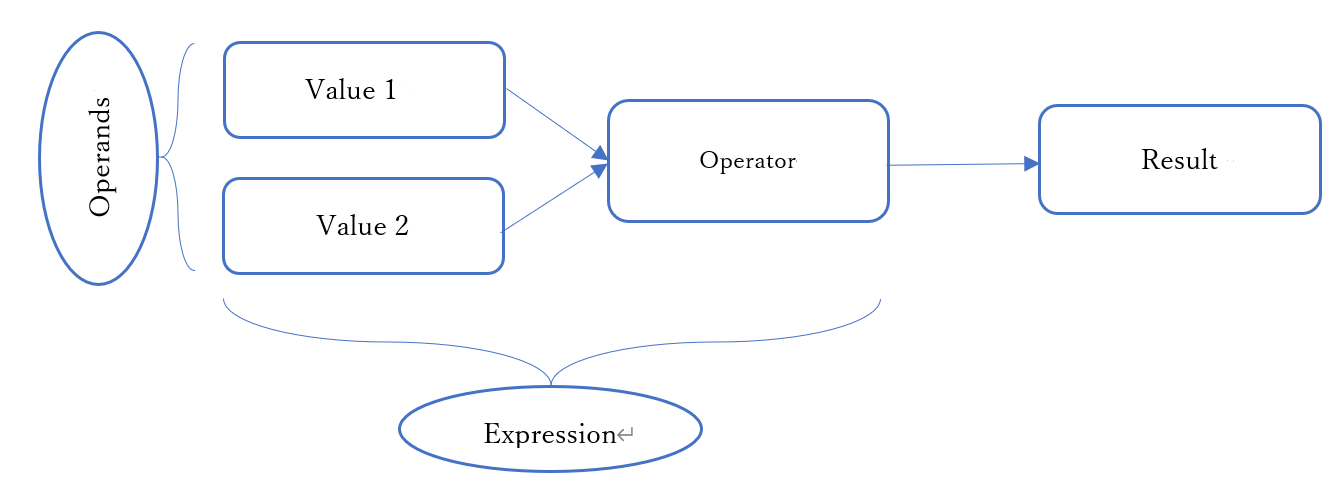## Types of operators

### Arithmetic Operators

For doing mathematical calculations on some operands, arithmetic operators are used.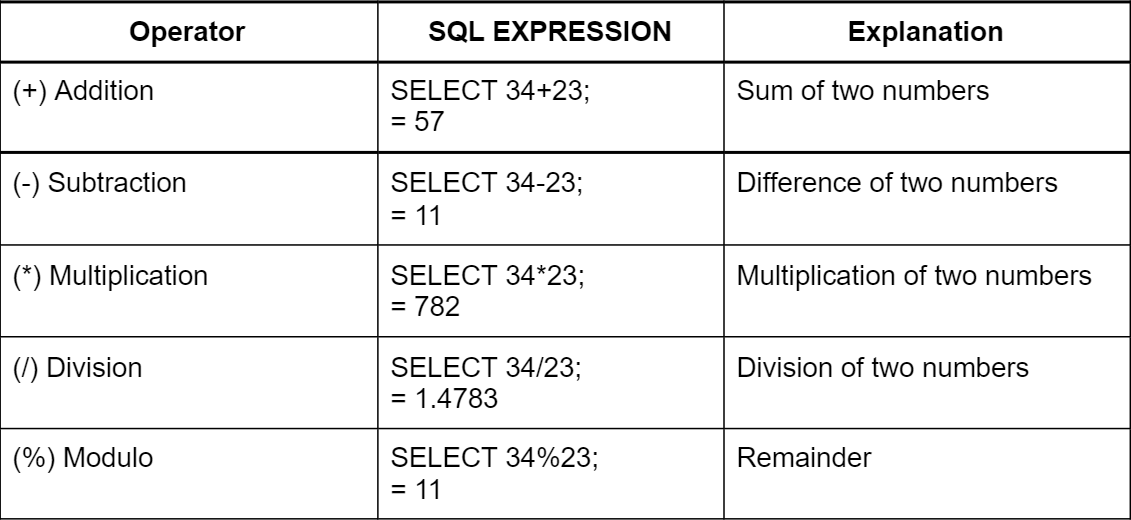### Comparison Operators

They are used to compare the operands and give results in the form of True(1) or False(0).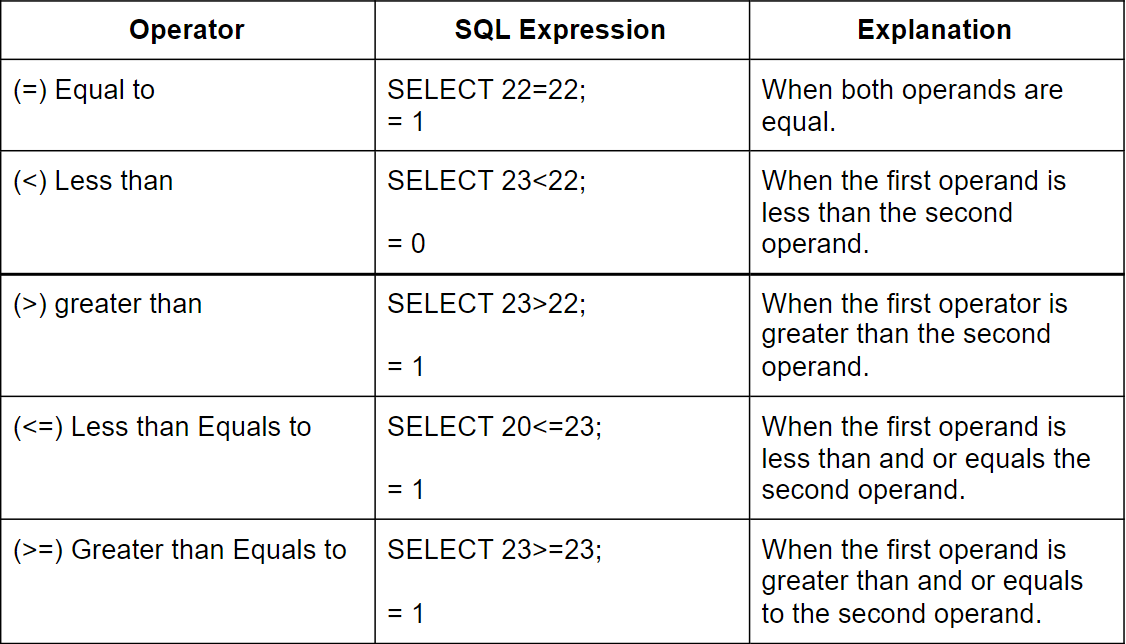### Bitwise Operators

It means manipulating a bit string, a bit array, or a binary number (also referred to as a bit string) at the bit level by bitwise operation. There are no special skills needed.

The result is obtained by comparing each bit of the first operand with its corresponding bit on the second operand. As we know, everything is in the form of bits, i.e., 1 or 0 combinations.

In this first, the operands are converted into the binary form:

Example:

34 - 100010

54 - 110110

Therefore if we use AND operation then:

``````1 0 0 0 1 0
1 1 0 1 1 0
AND
1 0 0 0 1 0 => 34``````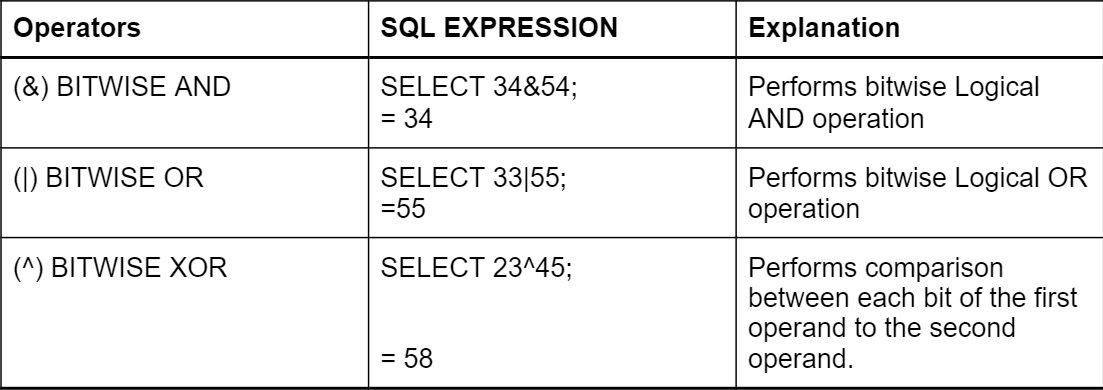### Logical Operators

These expressions combine two or more Boolean expressions so that the result of the compound expression will depend on the result of the original expression and the meaning of the operators. These expressions result in True(1) or False(0).

There are many operators in SQL. Let’s have a look at some of them.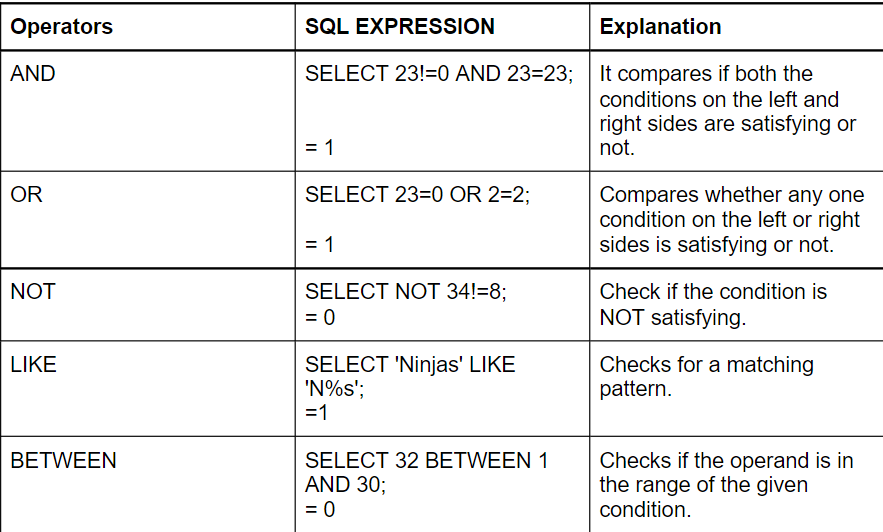Some more LIKE Operators :

• %N: Searches for a value that ends with ‘N.’
• N%: Searches for a value that starts with ‘N.’
• N_____: Searches for a value that starts with ‘N’ and has a length of 5 characters.
• N%s: Searches for a value that begins with ‘N’ and ends with ‘s.’
• %in%: Searches for a value that has ‘in’ at someplace.

Let’s see other Logical operators using the Database.

TABLE1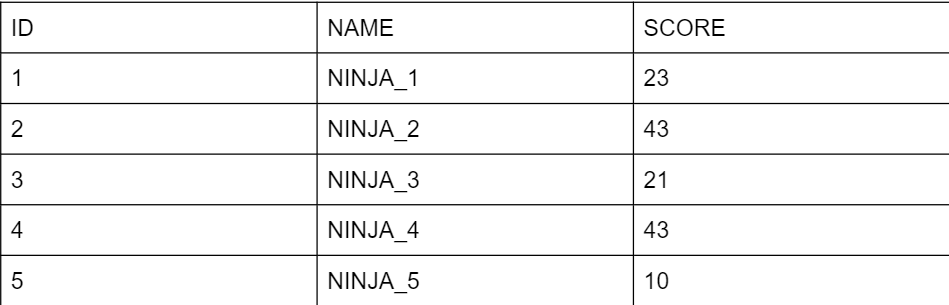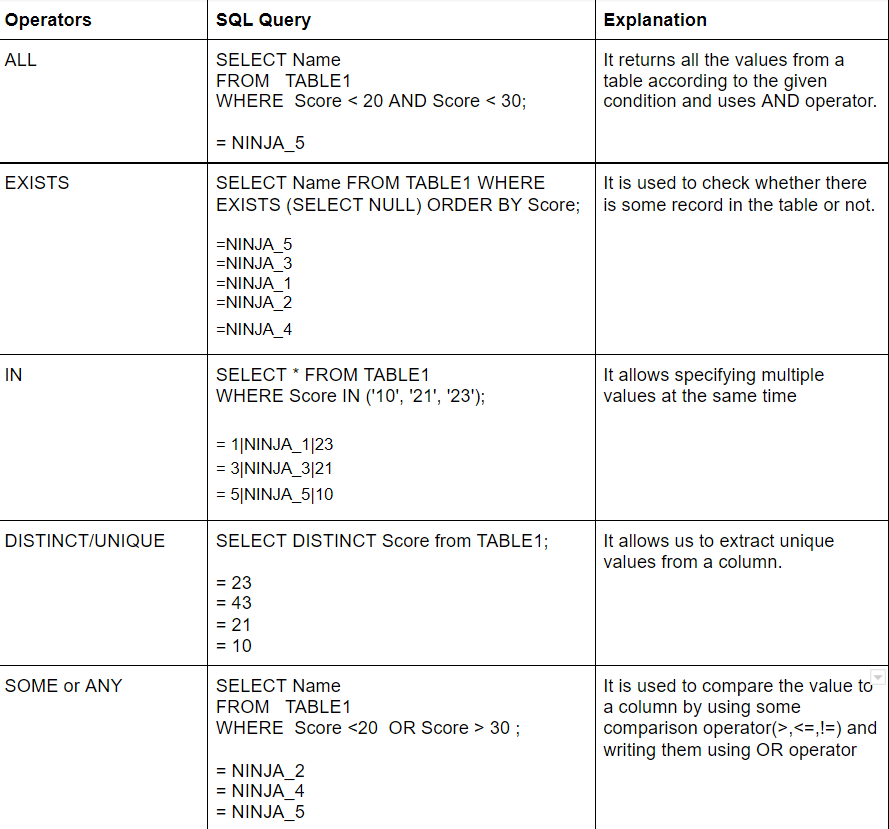Q1. How many types of operators are in SQL?

• There are six types of SQL operators:
• Arithmetic
• Bitwise
• Comparison
• Logical

Q2. Is Null an SQL operator?
The NULL operator determines if a column contains null values. The NULL operator filters the result set if a column contains null values.

Q3. What does the LIKE operator do in SQL?
This SQL Like operation determines whether a specified character string matches a given pattern. To find a particular pattern in a column, it is commonly used in a Where clause.

Q4. What is the difference between ‘IN’ and ‘BETWEEN’ operators?

• By using the BETWEEN operator, you can select a range of values between two values. Numeric values, text values, etc., can be used.
• Multiple values can be specified with the IN operator.

Q5. Can we use an operator for character data?
The SQL BETWEEN operator will return records whose column name contains any letter between character string values when we use it with the character values. In other words, if we use the NOT operator with the BETWEEN operator, then it will return all records whose column names do not begin between character values.

## Key Takeaways

In this blog, we have learned to use different operators in SQL. SQL operators help clean data and prepare it for analysis as part of data analysis. When a user requests data that fulfills some conditions, we use queries to transact data. The operators in the database make it possible for us to obtain the desired data values as efficiently and orderly as possible from the table records within the database.